# KR101674415B1 - Mehtod and apparatus for controlling signal gain in digital signal processing system - Google Patents

Mehtod and apparatus for controlling signal gain in digital signal processing system Download PDF

## Info

Publication number
KR101674415B1
KR101674415B1 KR1020120044582A KR20120044582A KR101674415B1 KR 101674415 B1 KR101674415 B1 KR 101674415B1 KR 1020120044582 A KR1020120044582 A KR 1020120044582A KR 20120044582 A KR20120044582 A KR 20120044582A KR 101674415 B1 KR101674415 B1 KR 101674415B1
Authority
KR
South Korea
Prior art keywords
power
period
input signal
data
signal
Prior art date
Application number
KR1020120044582A
Other languages
Korean (ko)
Other versions
KR20130121411A (en
Inventor
최경국
Original Assignee
삼성전자주식회사
Priority date (The priority date is an assumption and is not a legal conclusion. Google has not performed a legal analysis and makes no representation as to the accuracy of the date listed.)
Filing date
Publication date
Application filed by 삼성전자주식회사 filed Critical 삼성전자주식회사
Priority to KR1020120044582A priority Critical patent/KR101674415B1/en
Publication of KR20130121411A publication Critical patent/KR20130121411A/en
Application granted granted Critical
Publication of KR101674415B1 publication Critical patent/KR101674415B1/en

• 230000001276 controlling effects Effects 0 title 1

## Images

•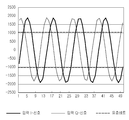•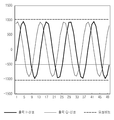•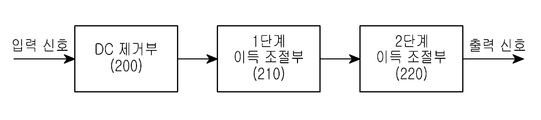•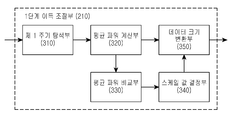•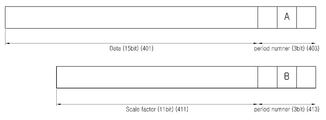•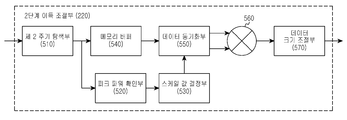•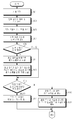•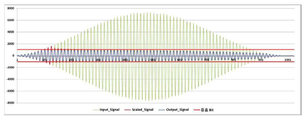•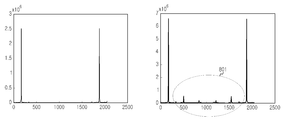•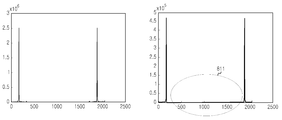## Classifications

• HELECTRICITY
• H03BASIC ELECTRONIC CIRCUITRY
• H03GCONTROL OF AMPLIFICATION
• H03G3/00Gain control in amplifiers or frequency changers without distortion of the input signal
• H03G3/20Automatic control
• HELECTRICITY
• H03BASIC ELECTRONIC CIRCUITRY
• H03GCONTROL OF AMPLIFICATION
• H03G3/00Gain control in amplifiers or frequency changers without distortion of the input signal
• H03G3/20Automatic control
• H03G3/30Automatic control in amplifiers having semiconductor devices
• H03G3/3036Automatic control in amplifiers having semiconductor devices in high-frequency amplifiers or in frequency-changers
• H03G3/3042Automatic control in amplifiers having semiconductor devices in high-frequency amplifiers or in frequency-changers in modulators, frequency-changers, transmitters or power amplifiers
• HELECTRICITY
• H03BASIC ELECTRONIC CIRCUITRY
• H03GCONTROL OF AMPLIFICATION
• H03G3/00Gain control in amplifiers or frequency changers without distortion of the input signal
• H03G3/20Automatic control
• H03G3/30Automatic control in amplifiers having semiconductor devices
• H03G3/3052Automatic control in amplifiers having semiconductor devices in bandpass amplifiers (H.F. or I.F.) or in frequency-changers used in a (super)heterodyne receiver
• H03G3/3078Circuits generating control signals for digitally modulated signals

## Abstract

A method of controlling a signal gain in a digital signal processing system includes the steps of measuring a peak power per cycle of an input signal and comparing the peak power and the threshold power per cycle And adjusting the signal amplitude of the period in which the peak power has a value greater than the threshold power so as to convert the power of the input signal into a signal in the valid bit and output the signal regardless of the power change of the input signal And stable signal characteristics can be obtained.

## Description

TECHNICAL FIELD  The present invention relates to a method and an apparatus for controlling a signal gain in a digital signal processing system,

The present invention relates to a digital signal processing system, and more particularly, to a signal gain control method and apparatus.

In a digital signal processing system in a mobile communication base station, a DDC (Digital Down Converter) for converting an input digital signal into a baseband signal and an AGC (Automatic Gain Controller) for converting the power of the converted baseband signal to a constant value are output And processes signals to be input to the modem. Here, the DDC maintains a generally high resolution in order to prevent signal distortion and maintain a high SNR (Signal Noise Ratio) characteristic. However, since the data required for signal demodulation in the modem actually has lower resolution than the output data of the DDC, the digital signal processing system extracts only the data corresponding to the valid bit from the output data of the DDC and provides it to the modem through the AGC. Therefore, the AGC performs the function of controlling the gain of the signal so that the power of the output signal always maintains a constant level regardless of the change of the power of the input signal.

FIG. 1A shows the relationship between the data signal output from the DDC and the data signal input to the AGC, and FIG. 1B shows the relationship between the output data signal of the AGC and the valid bit. Here, assuming that the output valid bit of the AGC is 11 bits, the range of the value that the valid bit can represent is 1023 to -1024. As shown in FIG. 1A, when the peak power of the data signal input to the AGC is about 1700, since the input signal is a signal that is not included in the output valid bit range, the AGC, as shown in FIG. It is possible to convert the signal into a signal included in the range of the valid bit and output it.

Conventionally, the AGC uses a method of adjusting an output signal using an average power. That is, the conventional AGC calculates the average power for the entire input signal and adjusts the size of the output signal based on the calculated average power. Such a method using the average power has a very long period for calculating and reflecting the average power. Therefore, when the power of the input signal changes instantaneously rapidly, it is difficult to recognize the instantaneous power change, It is difficult to control.

Also, in the method using the average power, when the power of the input signal is abruptly changed, the speed at which the average power is calculated with respect to the power change of the input signal is delayed, resulting in a loss of the signal and a clipping error There is a problem that can occur.

Therefore, an embodiment of the present invention is to provide a method and an apparatus for controlling a signal gain in a digital signal processing system.

Another embodiment of the present invention is to provide a method and apparatus for controlling a signal gain for each period according to peak power of a period of an input signal in a digital signal processing system.

Another embodiment of the present invention provides a method and apparatus for digitizing input signals in a digital signal processing system and controlling the size of an input signal based on a numbered period number.

According to another embodiment of the present invention, in the digital signal processing system, the first stage signal gain control is performed based on the average power of the input signal, and the second stage signal gain control is performed based on the peak power of the input signal A method and an apparatus.

According to an embodiment of the present invention, a method of controlling a signal gain in a digital signal processing system includes the steps of: measuring a peak power per cycle of an input signal; comparing peak power and a threshold power per cycle; And adjusting a signal size of a period having a value larger than the threshold power.

According to the embodiment of the present invention, in the digital signal processing system, the signal gain control apparatus measures the peak power per cycle of the input signal, compares the peak power per period with the threshold power, And a first gain adjuster for adjusting a signal amplitude of a period having a large value.

According to the present invention, a first-stage signal gain control is performed based on an average power of an input signal in a digital signal processing system, and a second-stage signal gain control is performed based on a peak power per cycle of an input signal, It is possible to convert the power of the input signal into a signal in the valid bit and output it regardless of the change, and stable signal characteristics can be obtained. In addition, it is possible to prevent the instability of the output signal due to the unstable signal input, thereby providing an effect of safely protecting the amplifier (AMP) or the RF (Radio Frequency) amplifying circuit.

1A is a diagram showing a relationship between a data signal output from the DDC and input to the AGC and a valid bit,
1B is a diagram showing the relationship between the output data signal of the AGC and the valid bit,
2 shows a simplified block diagram of an AGC in a digital signal processing system according to an embodiment of the present invention,
FIG. 3 is a block diagram showing a detailed block configuration of a one-stage gain adjuster constituting an AGC according to an embodiment of the present invention;
4 is a diagram illustrating numbering according to a period of an input signal in an AGC according to an exemplary embodiment of the present invention;
FIG. 5 is a block diagram showing a detailed block configuration of a two-stage gain adjusting unit configuring an AGC according to an embodiment of the present invention;
6 is a diagram illustrating a procedure for controlling a signal gain in an AGC according to an embodiment of the present invention;
7 is a graph showing a result of control of a signal gain of an AGC according to an embodiment of the present invention, and Fig.
8A and 8B are graphs showing input / output signals of an AGC according to the prior art and the present invention;

Hereinafter, preferred embodiments of the present invention will be described with reference to the accompanying drawings. In the following description of the present invention, a detailed description of known functions and configurations incorporated herein will be omitted when it may make the subject matter of the present invention rather unclear. In addition, the terms described below are defined in consideration of the functions of the present invention, which may vary depending on the intention of the user, the operator, or the custom. Therefore, the definition should be based on the contents throughout this specification.

Hereinafter, a method and an apparatus for controlling a signal gain in a digital signal processing system will be described.

FIG. 2 shows a simplified block diagram of an AGC in a digital signal processing system according to an embodiment of the present invention.

Referring to FIG. 2, the digital signal processing system includes a DC remover 200, a 1-stage gain adjuster 210, and a 2-stage gain adjuster 220.

The DC eliminator 200 removes the DC component of the input digital signal and provides it to the first stage gain adjuster 210. .

The first stage gain adjuster 210 determines a period of the input signal from the DC eliminator 200 and assigns a number indicating a period to each data constituting the input signal according to the determined period. The first stage gain adjuster 210 calculates the power of the input signal, measures the average power for a predetermined time, compares the measured average power with preset threshold power, and adjusts the size of the input signal according to the comparison result Or output the signal without adjusting the size of the input signal. Here, the average power refers to the average power of the input signal during a predetermined time interval rather than the average power over the entire input signal. The predetermined time interval for calculating the average power may be set by experiment. The predetermined threshold power means a power corresponding to the output valid bit of the AGC. For example, if the output valid bit of the AGC is n bits, then the threshold power may be 1023 (2 &lt; n &gt; -1).

The first stage gain adjuster 210 adjusts the magnitude of the input signal corresponding to the interval in which the average power is greater than the threshold power when the average power is greater than the threshold power. At this time, the first stage gain adjuster 210 determines a scale value (or a magnitude adjustment value) for the input signal according to the magnitude of the average power, and adjusts the magnitude of the input signal to a predetermined scale value. On the other hand, when the average power is less than or equal to the threshold power, the first-stage gain adjuster 210 outputs the input signal as it is, without changing the size, corresponding to a section in which the average power is less than or equal to the threshold power. Here, the first-stage gain control unit 210 determines whether the corresponding data is an input signal corresponding to an interval in which the average power is greater than the threshold power, based on the period number assigned to each data constituting the input signal Or whether the average power is an input signal corresponding to an interval in which the average power is kept smaller than or equal to the threshold power.

The two-stage gain adjuster 220 performs a function of finely adjusting a signal not adjusted to a signal within a range of valid bits in the one-stage gain adjuster 210 to be included in the valid bit range. That is, the two-stage adjuster 220 checks the peak power per cycle of the output signal from the one-stage gain adjuster 210, compares the peak power per cycle with a preset threshold power, The size of the signal can be adjusted by period, or the size of the input signal can be output without adjusting the period. At this time, the 2-stage gain controller 220 can check the peak power per cycle based on the period determined by the 1-stage gain controller 210 and the assigned period number. Here, the threshold power is equal to the threshold power used in the one-stage gain adjuster 210. That is, the threshold power used in the two-stage gain control unit 220 means the power corresponding to the output valid bit of the AGC.

The second stage gain adjuster 220 adjusts the magnitude of the input signal corresponding to the period when the peak power of the specific period is larger than the threshold power. At this time, the two-stage gain control unit 220 determines a scale value (or a magnitude adjustment value) for the input signal of the corresponding period according to the magnitude of the peak power, and determines the magnitude of the input signal corresponding to the corresponding period . On the other hand, when the peak power is smaller than or equal to the threshold power, the two-stage gain control unit 220 outputs the amplitude of the input signal corresponding to the period without adjustment. The two-stage gain control unit 220 determines whether the corresponding data is an input signal corresponding to a period longer than the threshold power, or whether the peak power is equal to or greater than a threshold value, based on the period number assigned to each data constituting the input signal. It is possible to judge whether the input signal corresponds to a period less than or equal to the power.

The one-stage gain control unit 210 and the two-stage gain control unit 220 shown in FIG. 2 will be described in detail with reference to FIGS. 3 to 5. FIG.

FIG. 3 shows a detailed block configuration of the one-stage gain control unit 210 constituting the AGC according to the embodiment of the present invention.

3, the one-stage gain adjuster 210 includes a first period searcher 310, an average power calculator 320, an average power comparator 330, a scale value determiner 340, And a conversion unit 350.

The first period search unit 310 receives an output signal from the DC removal unit 200 and determines a period based on the power of the input signal. At this time, the first period search unit 310 determines a period based on a point of time when the power of the input signal is converted from (-) power to (+) power or a point of time when the power of the input signal is converted into (-) power . More specifically, when data a having (-) power is input to the first period searching unit 310 and data b having (+) power is inputted immediately, the first period searching unit 310 divides the data b into n Th period. In addition, the first period search unit 310 calculates the power of the signal data inputted after the data b, and detects that the data d having the (+) power immediately after the data c having the (- , The data c can be determined to be the end of the n-th cycle and the data d can be determined to be the beginning of the (n + 1) -th cycle. For example, if the power of the signal data input to the first period search unit 310 is "-20, -10, 10, 20, 30, 20, 10, -10, -20, 20, -10, 10, 20, ..., 10, 20, ..., -20, -30, -20, -10 "may be determined as the n-th cycle, and then" 10,20, ... "

The first period search unit 310 determines a period of the input signal and then assigns a period number to each data constituting the input signal according to the determined period. At this time, the period number can be allocated by adding a predetermined number of bits to the bits of each data. That is, as shown in FIG. 4, the cycle number (A, 403) consisting of 3 bits can be added to the 15-bit data 401. For example, the first-stage gain adjuster 210 assigns a periodic number 1, which is a first period, to each of the data constituting the input signal of the first period, It is possible to assign cycle number 2, which means the second cycle, to each data. In other words, the first period search unit 310 assigns the same period number to all of the data constituting the input signal of a specific period, and outputs an I-component and a Quadrature-phase (Q-component) component, the same cycle number is assigned based on any one component. However, when signal processing of I and Q signals is separately performed, it is not necessary to assign the same periodic signal, and a unique periodic number is assigned according to each period. This allows the designer of the automatic gain control device to choose according to the characteristics of the system. In the first period search unit 310, the period number is assigned according to the period of the input signal. That is, in the first-stage gain control unit 210 and the second-stage gain control unit 210, So that the size thereof can be adjusted.

The average power calculator 320 calculates an average power for a predetermined time with respect to the input signal, provides the calculated average power to the average power comparator 330, and supplies the input signal to the data size converter 350 to provide. Here, the average power refers to the average power of the input signal during a predetermined time interval rather than the average power over the entire input signal. That is, the average power refers to an average power of a signal input during a previous predetermined time based on the current time point, and may be updated in a cycle unit of the input signal. For example, the average power calculator 320 calculates the average power of the signal of the nth period from the signal of the nt period at the input of the signal of the nth cycle, and calculates the average power of the signal of the It is possible to calculate the average power of the signal of the (n + 1) -th cycle from the signal of the (n-t + 1) -th cycle at the input timing. At this time, the predetermined time interval for calculating the average power can be set by experiment, and as the short time is set, it is possible to flexibly cope with the abrupt power change of the input signal. Since the power of the input signal is composed of (-) power and (+) power, the average power calculator 320 obtains the absolute value of each of the power of the input signal, Calculate the power.

The average power comparator 330 compares the average power calculated by the average power calculator 320 with a predetermined threshold power to determine whether the size of the input signal needs to be adjusted. When the average power is larger than the threshold power, the average power comparator 330 determines that the size of the input signal corresponding to the section where the average power is greater than the threshold power should be adjusted, Scale value determination unit 340 as shown in FIG. On the other hand, if the average power is smaller than or equal to the threshold power, the average power comparator 330 must output the input signal without changing the size, corresponding to the interval in which the average power is less than or equal to the threshold power And provides the determination result to the scale value determination unit 340.  Here, the scale information refers to information indicating whether the size is to be adjusted, a period number of the input signal corresponding to a section whose size is to be adjusted or not to be adjusted, and average power information. Here, the threshold power corresponds to the output valid bit of the AGC . For example, if the output valid bit of the AGC is n bits, then the threshold power may be 1023 (2 &lt; n &gt; -1).

When the scale information is provided from the average power comparator 330, the scale value determiner 340 determines a scale value according to the scale information and provides the determined scale value to the data size converter 350. [ At this time, the scale value determination unit 340 searches the scale value corresponding to the average power provided from the average power comparison unit 330 in the table indicating the previously stored scale value by average power, and assigns the cycle number to the searched scale value do. In this case, the period number means a period number included in the scale information, and may be allocated by adding a predetermined number of bits to a bit indicating a scale value. Here, the scale value may be a constant value capable of adjusting the overall power level of the input signal. That is, as shown in FIG. 4, a period number (B, 413) composed of 3 bits can be added to the 11-bit scale value 411. Here, by adding the period number to the scale value, the scale value can indicate the position of the signal to be applied. Here, when the scale information indicating that the scaling is not performed is input to the input signal of a specific period from the average power comparing unit 330, the scale value determining unit 340 determines a scale scale value You can decide. In this case, the predetermined basic scale value may be a value that does not change the signal size of the period even if it is applied to the signal of the corresponding period.

The data size conversion unit 350 adjusts the size of the input signal by applying the scale value from the scale value determination unit 340 to the input signal from the average power calculation unit 320. [ At this time, the data size conversion unit 350 multiplies the scale value by each of the data having the same cycle number as the scale value among the signals input from the average power calculation unit 320 after confirming the cycle number assigned to the scale value . Here, the input signal to which the scale value is applied is only adjusted in size while maintaining the phase. In addition, the data size conversion unit 350 may multiply the input signal by the scale value, and then change the number of bits increased by the multiplication operation to the original number of bits. For example, when the scale value is composed of n bits, the data size conversion unit 350 divides the data to which the scale value is applied by n bits, so that the resulting data has the same number of bits as the original data.

The data size conversion unit 350 outputs a signal whose magnitude is adjusted by the scale value to the second-stage gain adjustment unit 220.

FIG. 5 shows a detailed block configuration of a two-stage gain controller 220 constituting an AGC according to an embodiment of the present invention.

5, the two-stage gain control unit 220 includes a second period search unit 510, a peak power check unit 520, a scale value determination unit 530, a memory buffer 540, a data synchronization unit 550, a multiplier 560, and a data scaler 570.

The second period search unit 510 determines the period of the signal provided from the first stage gain adjuster 210. This is a process for checking the range of the periodic signal for determining the peak power per cycle. Since the signal provided from the first stage gain adjuster 210 is already allocated in the first stage gain adjuster 210 according to the period of the signal, the second period searcher 510 It does not perform the cycle number assignment operation of FIG.

The peak power check unit 520 calculates the peak power per signal cycle according to the period of the signal checked in the second period search unit 510. [ That is, the peak power check unit 520 confirms the power of each signal data having the same period number, determines the largest power value, and determines the peak power as the peak power. The peak power verifying unit 520 provides the peak power per period of the signal to the scale value determining unit 530. [

The scale value determining unit 530 receives the peak power of each cycle of the signal from the peak power checking unit 520 and compares the peak power and the threshold power according to the cycle of the signal to determine whether the size of the signal of each cycle needs to be adjusted . That is, when the peak power of the specific period is greater than the threshold power as a result of comparing the peak power of the specific period with the threshold power, the scale value determining unit 530 determines that the amplitude of the signal for the specific period needs to be adjusted, It is determined that it is not necessary to adjust the size of the signal with respect to the specific period when the peak power of the signal is less than or equal to the threshold power. Then, the scale value determining unit 530 determines a scale value for a signal of a period whose size needs to be adjusted. At this time, the scale value determining unit 530 may determine the scale value by referring to a table showing the previously stored scale value for each peak power, or may determine the scale value using a function for determining the scale value according to the peak power . Here, the scale value may be a constant value capable of adjusting the power level of the signal of the corresponding period.

In addition, the scale value determining unit 340 may determine that the amplitude of the signal is not needed for the signal of the period in which the peak power is equal to or less than the threshold power, and may determine that the predetermined basic scale value is applied regardless of the peak power . In this case, the predetermined basic scale value may be a value that does not change the signal size of the period even if it is applied to the signal of the corresponding period.

The scale value determining unit 530 may assign a period number indicating the corresponding period to the determined scale value when the scale value for the period in which the peak power exceeds the threshold power is determined. For example, as shown in FIG. 4, a 3-bit period number (B, 413) can be added to an 11-bit scale value 411. Here, by adding the period number to the scale value, the scale value can indicate the position of the signal to be applied.

The memory buffer 540 stores the data corresponding to each period output from the second period searching unit 510 while the scale power value determining unit 520 and the scale determining unit 530 determine the scale value corresponding to each period, . That is, when a signal of a specific period is input from the second period search unit 510, the memory buffer 540 temporarily stores a signal of a specific period until a scale value for a specific period is determined by the scale determination unit 530 .

The data synchronization unit 550 performs a function of synchronizing the synchronization between the data of the period of the input signal and the scale value corresponding to each period of the input signal. That is, in order to allow the multiplier 560 to multiply the data having the same period number and the scale value, the data synchronizer 550 multiplies the data of each period of the input signal and the scale value corresponding to each period of the input signal I am motivated for. At this time, the data synchronization unit 550 performs synchronization so that a scale value having the same period number can be calculated for a plurality of data having the same period number.

The multiplier 560 multiplies the data having the same period number output from the data synchronization unit 550 by the scale value and provides the result to the data size adjustment unit 570.

The data size adjustment unit 570 performs a function for changing the number of bits increased by the multiplication operation of the multiplier 560 to the original number of bits. For example, when the scale value is composed of n bits, the data size adjuster 570 divides the result of multiplying the scale value by n bits, and the final result data is the same bit as the number of bits of the original data Number.

3 and 5, when the first period search unit 310 and the second period search unit 510 are included in the first-stage gain adjuster 210 and the second-stage gain adjuster 220, respectively, The first period search unit 310 and the second period search unit 510 may be configured separately from the first stage gain adjuster 210 and the second stage gain adjuster 220, respectively.

In FIGS. 3 and 5, all the signals pass through the multiplier, so that the magnitude of the signal is not multiplied by the base scale value even though the signal is not required to be changed in size. However, according to the design method, For the signal, the multiplier may be bypassed to prevent the scale value from being applied.

FIG. 6 illustrates a procedure for controlling a signal gain in an AGC according to an embodiment of the present invention.

Referring to FIG. 6, in step 601, the AGC receives an output signal from a digital down converter (DDC). At this time, the AGC can remove the DC component of the input signal.

In step 603, the AGC determines the power and period of the input signal. That is, the AGC measures the power of each data constituting the input signal and determines the period of the input signal based on the measured power. Here, the AGC determines the period based on a point of time when the power of the input signal is converted from (-) power to (+) power or a point of time when the input signal is converted from (+) power to (-) power. More specifically, the AGC can determine the data b as the start of the n-th period when the data a having the (-) power is input and the data b having the (+) power is input. Also, the AGC calculates the power of the signal data inputted after the data b, and when the data c having the (-) power is inputted and the data d having the (+) power is inputted, Th cycle, and the data d can be determined as the beginning of the (n + 1) -th cycle.

Thereafter, the AGC proceeds to step 605 and adds a period number to each data constituting the input signal according to the determined period. That is, as shown in FIG. 4, the AGC can add a cycle number (A, 403) consisting of 3 bits to 15-bit data 401. For example, the AGC assigns the cycle number 1, which means the first cycle, to each of the plurality of data constituting the input signal of the first cycle, and the second cycle is assigned to each of the plurality of data constituting the input signal of the second cycle. Quot; 2 &quot; That is, the AGC assigns the same period number to all the data constituting the input signal of a specific period, and furthermore, for the I-component and the Q-component (quadrature-phase component) The same period number is assigned based on any one of the components. Here, the period number is assigned according to the period of the input signal in order to adjust only the signal of the period satisfying the condition according to the embodiment of the present invention.

In step 607, the AGC determines the average power of the input signal for a predetermined time. In step 609, the AGC compares the average power with the threshold power to determine whether the average power is greater than the threshold power. If the average power is less than or equal to the threshold power, the AGC proceeds directly to step 615 below.

On the other hand, if the average power is greater than the threshold power, the AGC determines the first scale value based on the average power in step 611. [ Here, the AGC can determine the scale value based on the table showing the previously stored scale value by the average power. In order to indicate the position of the signal to which the determined scale value is applied, as shown in FIG. 4, . In this case, the period number may be a period number of the input signal corresponding to an interval in which the average power is larger than the threshold power.

In step 613, the AGC adjusts the magnitude of the input signal corresponding to the interval in which the average power is larger than the threshold power, using the first scale value. That is, the AGC multiplies the input signal corresponding to the section where the average power is larger than the threshold power by the first scale value, and adjusts the size only while maintaining the phase of the input signal. At this time, whether AGC is an input signal corresponding to an interval in which the average power is larger than the threshold power may be determined by using a period number assigned to each data constituting the input signal.

Thereafter, the AGC proceeds to step 615 and determines whether the peak power per cycle is greater than the threshold power. For a period in which the peak power has a value less than or equal to the threshold power, the AGC proceeds to step 617 and outputs the signal of the corresponding period without additional scale, that is, without adjusting the size of the additional signal.

On the other hand, for a period in which the peak power has a value larger than the threshold power, the process proceeds to step 619 to determine a second scale value for the period. In step 621, the signal intensity of the period is adjusted to a second scale value And outputs it. Here, the AGC can determine a scale value based on a table indicating a scale value for each peak power or a function for a scale value for each peak power. The AGC also assigns a period number to the scale value, as shown in FIG. 4, to indicate the position of the signal to which the determined scale value is to be applied. At this time, the period number may be a period number of a period whose peak power is larger than the threshold power. Also, the AGC multiplies the second scale value by a signal of a period whose peak power is larger than the threshold power, and adjusts the size only while maintaining the phase of the input signal. At this time, the AGC can determine the data corresponding to the period to which the second scale value is applied based on the period number assigned to the second scale value.

Thereafter, the AGC returns to step 601 and re-executes the following steps.

7 is a graph showing a result of controlling the signal gain of the AGC according to the embodiment of the present invention. 7 shows the relationship between the input signal and the output signal when power is calculated using only the I component among the input signals in the AGC according to the present invention. As shown in FIG. 7, the AGC according to the present invention can change the input signal so as to be included within the valid bit range and output it even if the power of the input signal increases rapidly.

8A and 8B show graphs showing input / output signals of AGC according to the prior art and the present invention.

FIG. 8A shows an input signal and an output signal of the AGC according to the prior art in the frequency domain, and FIG. 8B shows an input signal and an output signal of the AGC according to the present invention in the frequency domain.

As shown in FIG. 8A, the AGC according to the prior art can recognize that a noise component that was not present in the input signal is generated in the output signal. On the other hand, as shown in FIG. 8B, it can be seen that the AGC according to the present invention outputs the same signal as the input signal without signal distortion.

In the present invention, for convenience of description, when the average power or the peak power is larger than the threshold power, a method of adjusting the size of the signal of the period to be included in the power of the signal of the period, . However, according to the embodiment of the present invention, two threshold powers having different values are set, and when the average power or the peak power is smaller than the threshold power by a certain value or more, The present invention can be applied to a method of largely adjusting the signal amplitude of the period.

While the present invention has been described in connection with what is presently considered to be the most practical and preferred embodiment, it is to be understood that the invention is not limited to the disclosed embodiments. Therefore, the scope of the present invention should not be limited by the illustrated embodiments, but should be determined by the scope of the appended claims and equivalents thereof.

## Claims (20)

1. A method for controlling a signal gain in a digital signal processing system,
Determining a period of each data based on power of each data constituting an input signal;
Allocating a period number to each piece of data by adding at least one bit indicating a period number to each piece of data based on the determined period;
Measuring a peak power per cycle of the input signal based on a period number added to each data;
Comparing the peak power and the threshold power per cycle,
Adjusting a magnitude of each data corresponding to a period in which the peak power has a value larger than the threshold power in the input signal.
2. delete
3. The method according to claim 1,
Wherein the step of measuring the peak power of each period of the input signal comprises:
And determining a maximum power among the powers of the data to which the same period number is added as the peak power.
4. 4. The method of claim 3, further comprising: measuring an average power of the input signal before measuring a peak power of the input signal by a period;
Comparing the average power with the threshold power;
Adjusting the magnitude of the input signal if the average power is greater than the threshold power and not adjusting the magnitude of the input signal if the average power is less than or equal to the threshold power.
5. The method of claim 3,
Wherein the step of adjusting the size of each data corresponding to the period in which the peak power has a value larger than the threshold power,
Determining a scale value according to the peak power;
Assigning a period number of a period in which the peak power is greater than the threshold power to the scale value;
A multiplier for multiplying a scale value having the same period number and data based on the period number assigned to the scale value and the period number added to the data to calculate a data corresponding to a period whose peak power is greater than the threshold power Are included within the range of significant bits.
6. 6. The method of claim 5,
Wherein the step of assigning the period number of the period in which the peak power has a value larger than the threshold power to the scale value,
And adding at least one bit indicating a period number of a period in which the peak power has a value larger than the threshold power to the scale value,
Wherein the step of adjusting data included in the range of valid bits of the data corresponding to the period in which the peak power has a value larger than the threshold power comprises the step of performing a division operation on the data obtained by the multiplication operation, &Lt; / RTI &gt;

7. The method according to claim 1,
Wherein the step of determining the period of each data based on the power of each data constituting the input signal comprises:
A process of determining the period based on any one of a time when the power of each data constituting the input signal is converted from (-) power to (+) power or a time when (+) power is converted into (- &Lt; / RTI &gt;
8. The method according to claim 1,
Wherein the step of adding the period number to each of the data based on the determined period comprises:
And adding the same period number to the I component and the Q component constituting the input signal.
9. The method according to claim 1,
Further comprising the step of outputting, in the input signal, each data corresponding to a period in which the peak power has a value smaller than or equal to the threshold power, without scaling.
10. The method according to claim 1,
Estimating a direct current (DC) of the input signal before measuring a peak power per cycle of the input signal;
And removing the estimated DC.
11. A signal gain control apparatus in a digital signal processing system,
Determining a period of each data based on the power of each data constituting the input signal and adding at least one bit indicating a period number to each data based on the determined period to assign a period number to each data ; And
The peak power of each period of the input signal is measured based on the period number added to each data, and the peak power per period is compared with the threshold power, and when the peak power is larger than the threshold power And a first gain adjuster for adjusting a magnitude of each data corresponding to a period of the first period.
12. delete
13. 12. The method of claim 11,
Wherein the first gain control unit determines the maximum power among the powers of the data to which the same period number is added as the peak power.
14. 14. The method of claim 13, wherein the first gain controller measures an average power of the input signal before measuring the peak power of the input signal by the period, compares the average power with the threshold power, Further comprising a second gain adjuster that adjusts the magnitude of the input signal if the average power is greater than or equal to the threshold power and does not adjust the magnitude of the input signal if the average power is less than or equal to the threshold power.
15. 14. The method of claim 13,
Wherein the first gain controller determines a scale value corresponding to the peak power and assigns a period number of a period having a peak power greater than the threshold power to the scale value, And multiplying the scaled value having the same period number by the data based on the period number added to each data so that the data corresponding to the period having the peak power greater than the threshold power are included in the range of the valid bit Adjusting device.
16. 16. The method of claim 15,
Wherein the first gain control unit adds at least one bit indicating a period number of a period in which the peak power has a value larger than the threshold power to the scale value, A device that divides by a value composed of the same bits.
17. 12. The method of claim 11,
Wherein the period search unit is configured to determine whether the power of each of the data constituting the input signal is converted from (-) power to (+) power or from a (+) power to A device for determining a period.
18. 12. The method of claim 11,
Wherein the period search unit adds the same period number to the I component and the Q component that constitute the input signal based on the determined period.
19. 12. The method of claim 11,
Wherein the first gain controller outputs each data corresponding to a period in which the peak power is smaller than or equal to the threshold power in the input signal without size adjustment.
20. 12. The method of claim 11,
Further comprising a DC elimination section for estimating DC (Direct Current) of the input signal and for removing the estimated DC before measuring the peak power per cycle of the input signal.
KR1020120044582A 2012-04-27 2012-04-27 Mehtod and apparatus for controlling signal gain in digital signal processing system KR101674415B1 (en)

## Priority Applications (1)

Application Number Priority Date Filing Date Title
KR1020120044582A KR101674415B1 (en) 2012-04-27 2012-04-27 Mehtod and apparatus for controlling signal gain in digital signal processing system

## Applications Claiming Priority (2)

Application Number Priority Date Filing Date Title
KR1020120044582A KR101674415B1 (en) 2012-04-27 2012-04-27 Mehtod and apparatus for controlling signal gain in digital signal processing system
US13/873,130 US9106195B2 (en) 2012-04-27 2013-04-29 Method and apparatus for controlling signal gain in digital signal processing system

## Publications (2)

Publication Number Publication Date
KR20130121411A KR20130121411A (en) 2013-11-06
KR101674415B1 true KR101674415B1 (en) 2016-11-09

# Family

## Family Applications (1)

Application Number Title Priority Date Filing Date
KR1020120044582A KR101674415B1 (en) 2012-04-27 2012-04-27 Mehtod and apparatus for controlling signal gain in digital signal processing system

## Country Status (2)

US (1) US9106195B2 (en)
KR (1) KR101674415B1 (en)

## Families Citing this family (1)

* Cited by examiner, † Cited by third party
Publication number Priority date Publication date Assignee Title
CN106817719B (en) * 2017-02-06 2019-07-16 上海鸿洛通信电子有限公司 TRP test method and device based on tdd mode

## Citations (3)

* Cited by examiner, † Cited by third party
Publication number Priority date Publication date Assignee Title
US20010043118A1 (en) * 2000-05-18 2001-11-22 Ntt Docomo, Inc. Feed-forward amplifier
US20030036374A1 (en) * 2001-06-04 2003-02-20 Time Domain Corporation Wireless local area network using impulse radio technology to improve communications between mobile nodes and access points
US20100067587A1 (en) * 2008-09-18 2010-03-18 Raytheon Company Adaptive pulse detection for all-digital line-of-sight (los) processor

## Family Cites Families (10)

* Cited by examiner, † Cited by third party
Publication number Priority date Publication date Assignee Title
FR2542540B1 (en) * 1983-03-08 1989-02-10 Canon Kk image processing system
US5117384A (en) * 1990-01-24 1992-05-26 International Business Machines Corporation Method and apparatus for exponent adder
US5081645A (en) * 1990-08-06 1992-01-14 Aware, Inc. Novel spread spectrum codec apparatus and method
US6052371A (en) * 1996-03-14 2000-04-18 Telefonaktiebolaget L M Ericsson (Publ) System and method for the communication of operation and maintenance, administration and provisioning information over an asynchronous transfer mode network
US7079161B2 (en) * 2001-06-14 2006-07-18 Canon Kabushiki Kaisha Image display apparatus
PT2271111T (en) * 2001-11-06 2016-11-08 Panasonic Ip Corp America Moving picture coding method and moving picture decoding method
JP4249412B2 (en) * 2001-12-27 2009-04-02 Ｎｅｃエレクトロニクス株式会社 Semiconductor memory device
KR20050062866A (en) 2003-12-19 2005-06-28 엘지전자 주식회사 Method for calculation gain dagc of dmb receiver
US8154995B2 (en) * 2005-01-26 2012-04-10 At&T Intellectual Property I, L.P. System and method of managing digital data transmission
US8548105B2 (en) 2008-08-05 2013-10-01 Qualcomm Incorported Joint time-frequency automatic gain control for wireless communication

## Patent Citations (3)

* Cited by examiner, † Cited by third party
Publication number Priority date Publication date Assignee Title
US20010043118A1 (en) * 2000-05-18 2001-11-22 Ntt Docomo, Inc. Feed-forward amplifier
US20030036374A1 (en) * 2001-06-04 2003-02-20 Time Domain Corporation Wireless local area network using impulse radio technology to improve communications between mobile nodes and access points
US20100067587A1 (en) * 2008-09-18 2010-03-18 Raytheon Company Adaptive pulse detection for all-digital line-of-sight (los) processor

## Also Published As

Publication number Publication date
US20130287079A1 (en) 2013-10-31
US9106195B2 (en) 2015-08-11
KR20130121411A (en) 2013-11-06

## Similar Documents

Publication Publication Date Title
CN101427459B (en) Method and arrangement for optimizing efficiency of a power amplifier
JP4905344B2 (en) Power amplifier
DE60209088T2 (en) Method and device for continuous control of the dynamic range of an analog-to-digital converter
EP2386144B1 (en) Circuits, systems, and methods for managing automatic gain control in quadrature signal paths of a receiver
JP3844352B2 (en) Transmitter
KR101693610B1 (en) Systems and methods for distributed antenna system reverse path summation using signal-to-noise ratio optimization
JP4589443B2 (en) Method and apparatus for DC offset correction in a digital-to-analog converter
JP4497470B2 (en) High frequency power amplifier and transmitter
JP4842973B2 (en) Closed loop power control with high dynamic range
KR101636016B1 (en) Apparatus for receiving signal and compensating phase mismatch method thereof
JP5365369B2 (en) Transmission apparatus, distortion compensation apparatus, and distortion compensation method
DE19835418B4 (en) Messaging device for digital messaging
JP2014535214A (en) Block-based crest factor reduction (CFR)
KR101236018B1 (en) Method and system for controlling the gain of an amplifier by continuously alternating closed and open loop power control
US20050174274A1 (en) Method and system for mixed analog-digital automatic gain control
JP6116865B2 (en) Apparatus and method for correcting output characteristics in power coupling device
WO2007077838A1 (en) Polar coordinate modulated transmission device, adaptive distortion compensation processing system, polar coordinate modulated transmission method, and adaptive distortion compensation processing method
US7280812B2 (en) Digital baseband receiver with DC discharge and gain control circuits
JP2005348440A (en) Method and apparatus for automatic gain control
JP2009177640A (en) Power amplifier
KR101083944B1 (en) Adaptive CFR according to average power variation of input signal and method thereof
CN102790620B (en) Having a digital-analog conversion device depends on the power range of d / a converter selected
KR100527000B1 (en) Automatic gain control apparatus capable of compensating dc offset in orthogonal frequency division multipexing and method thereof
US7583809B2 (en) Sound signal processing device and sound signal processing method

## Legal Events

Date Code Title Description
A201 Request for examination
E902 Notification of reason for refusal
E701 Decision to grant or registration of patent right
GRNT Written decision to grant
FPAY Annual fee payment

Payment date: 20191030

Year of fee payment: 4